## 基本数值的增长

### 生命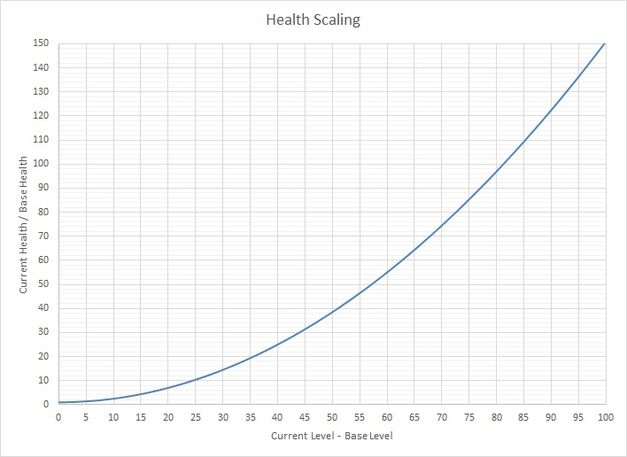### 护盾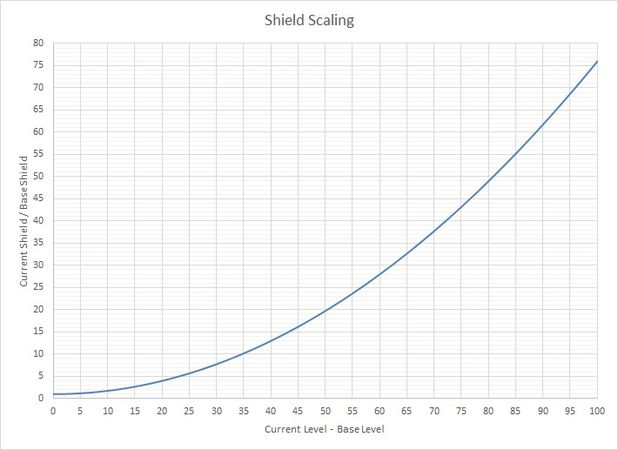### 护甲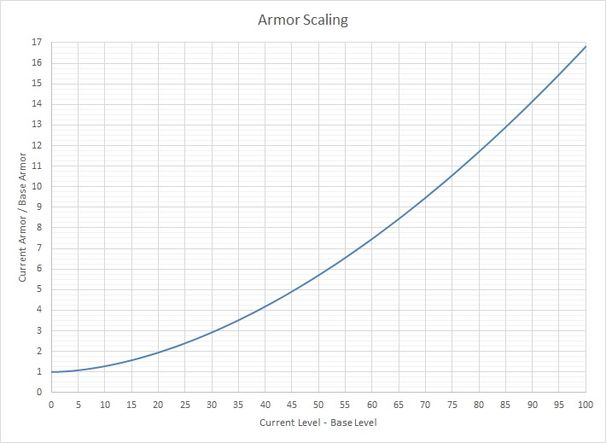### 伤害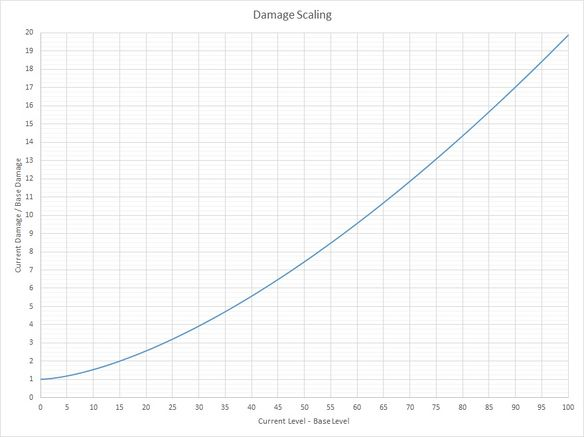### 经验值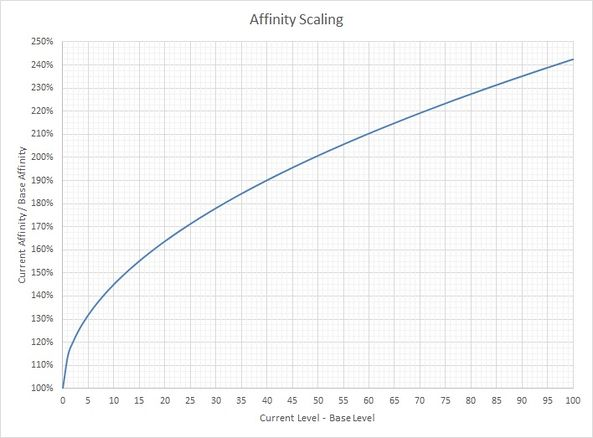## 派生数值的增长规律

### 有效生命值

#### 对于有生命和护盾的敌人#### 对于有生命和护甲的敌人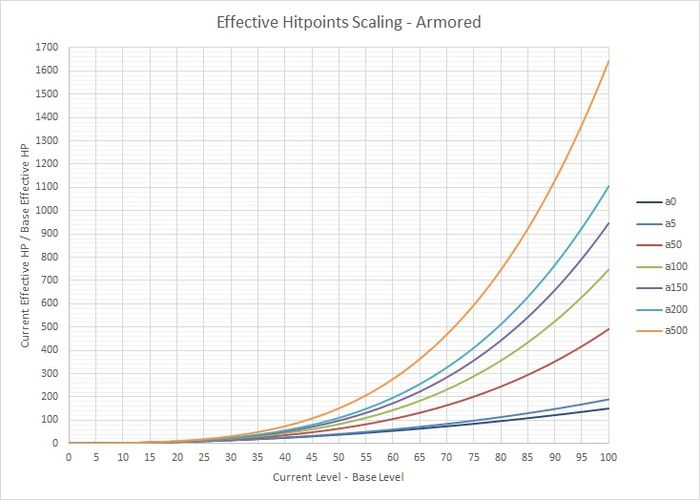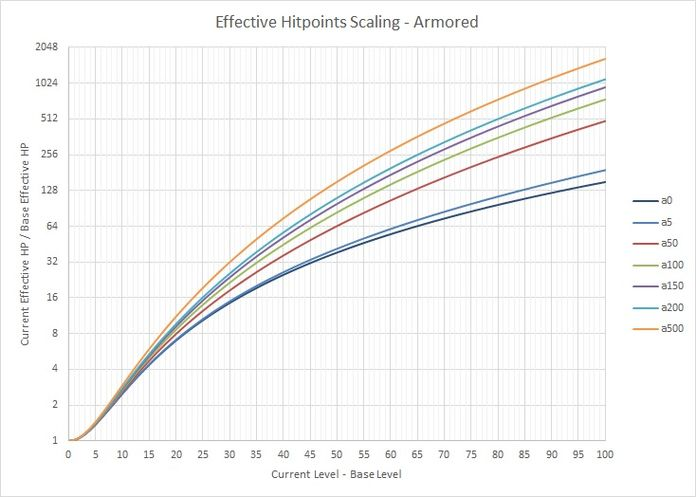### 护盾比例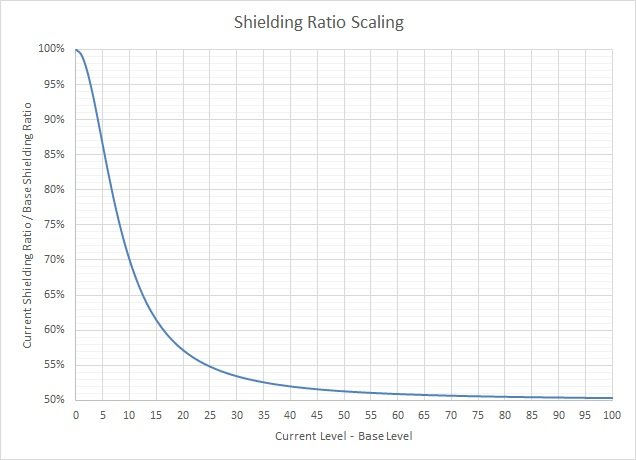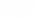$\qquad H_b=$基础生命值（是个常数）
$\qquad H_c=$当前生命值
$\qquad S_b=$基础护盾值（是个常数）
$\qquad S_c=$当前护盾值
$\qquad L_b=$基础等级（是个常数）
$\qquad L_c=$当前等级
$\qquad R_b=$基础比例（是个常数）
$\qquad R_C=$当前比例

$$R_b=\frac{S_b}{H_b} \\$$ 从前面给出的方程，我们能列出：
$$S_c=S_b\times\left[1+{\left(L_c-L_b\right)}^{2}\times 0.0075\right] \\$$ $$H_c=H_b\times\left[1+{\left(L_c-L_b\right)}^{2}\times 0.015\right] \\$$ 所以很明显，如果我们想要得到当前比例，我们只需要：
$$R_c=\frac{S_c}{H_c}=\frac{S_b\times\left[1+{\left(L_c-L_b\right)}^{2}\times 0.0075\right]}{H_b\times\left[1+{\left(L_c-L_b\right)}^{2}\times 0.015\right]}\\$$ 然后利用自变量趋近$+\infty$来得到在敌人等级无穷大时的比例趋近值：
$$\lim_{L_c\rightarrow+\infty}{R_c}=\lim_{L_c\rightarrow+\infty}{\left(\frac{S_b\times\left[1+{\left(L_c-L_b\right)}^{2}\times 0.0075\right]}{H_b\times \left[1+{\left(L_c-L_b\right)}^{2}\times 0.015\right]}\right)}\\$$ 由于$L_b, S_b, H_b$均为常数，反复使用洛必达法则就能写出答案：
\begin{align} \lim_{L_c\rightarrow+\infty}{R_c}= &\lim_{L_c\rightarrow+\infty}{\left(\frac{S_b\times\left[1+{\left(L_c-L_b\right)}^{2}\times 0.0075\right]}{H_b\times \left[1+{\left(L_c-L_b\right)}^{2}\times 0.015\right]}\right)}\\ &=\frac{S_b}{H_b}\times \lim_{L_c\rightarrow+\infty}{\frac{1+{\left(L_c-L_b\right)}^{2}\times 0.0075}{1+{\left(L_c-L_b\right)}^{2}\times 0.015}}\\ &=R_c \times \lim_{L_c\rightarrow+\infty}{\frac{0.0075\times 2 \times L_c-0.0075\times 2\times L_b}{0.015 \times 2 \times L_c-0.015\times 2 \times L_b}}\\ &=R_c \times \lim_{L_c\rightarrow+\infty}{\frac{0.0075\times2}{0.015\times2}}\\ &=R_c \times \frac{1}{2}\\ \end{align} 最终：
$$\lim_{L_c\rightarrow+\infty}{R_c}=\frac{R_b}{2}$$

• 这个结论意味着，当当前等级（$L_c$）无尽趋近于无穷大时，当前护盾比例（$R_b$）等于基础护盾比例的一半。也就是说，等于100%的一半：50%。

### 经验值密度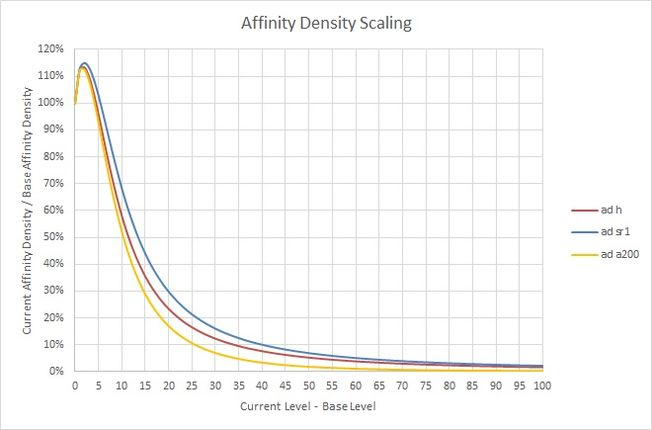### 反射击杀率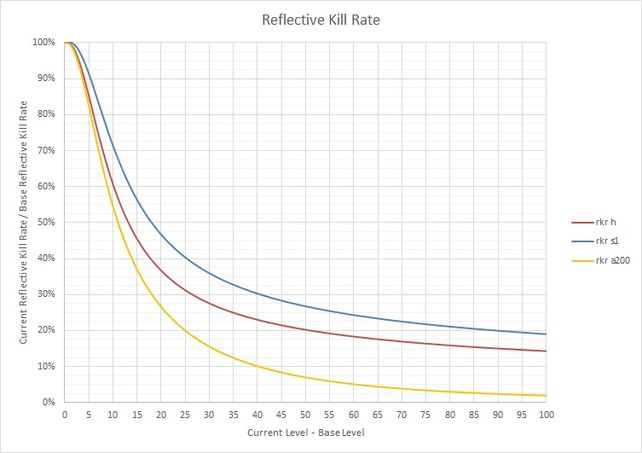## 公式的现状

Warframe的增长规律公式以前已经受到暗改的影响，即补丁说明中没有提及，就像伤害公式一样。 他们可能不时需要重新验证。

0.0
0人评价
0

11个月
Kinrtoki
-2

1年
Chaldea
0

1年